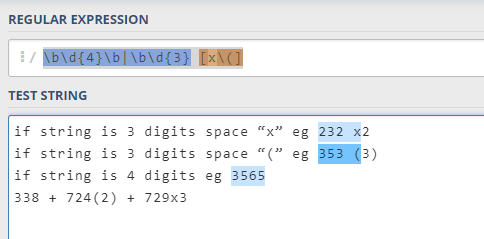# Regarding regex

Hi,
condition1
I want to extract 3 digit number from input which could be delimited by space,comma, or semicolon.
eg-

input-343,232
output-343 232

condition 2
Also if input is like 232(2) or 343x3
The output should be x=232 and in other variable lets say y=2
and x-343 y=3

So both condition should be satisfied.

\d{3} this will give you three digit
if you want (2) and 3 you can use this (?<=d{3}.).[0-9]*
you can use this!
@shanewatson

`(?P<x>\b\d{3})[\(x](?P<y>\d+)|(?P<simple>\d{3})\b`

I want to check 3 conditions:-

1. if string is 3 digits space “x” eg 232 x2
2. if string is 3 digits space “(” eg 353 (3)
3. if string is 4 digits eg 3565

i was using something like this,could you help on this

\d{3} x|\d{3} (([^)]+))|\d{4}

if string has 4 digits??
wht you want to pick??

(?<=\d{3}\s.).[0-9]*
try this!
Cheers
@shanewatson

if either condition is true…i want to result is out as boolean:true

1 Like

use IsMatches Activity then!

for this input string : 338 + 724(2) + 729x3

it should result as false,but it is matching

which activity you are using?

1 Like

I’m afraid I might not understand clearly your problem but the following will check if your string contains a part complying to at least one of the three conditions.

`\b\d{4}\b|\b\d{3} [x\(]` or, if you don’t mind the groups, `\b\d{3}(\d\b| [x\(])`did you get the result as expected?
@shanewatson